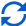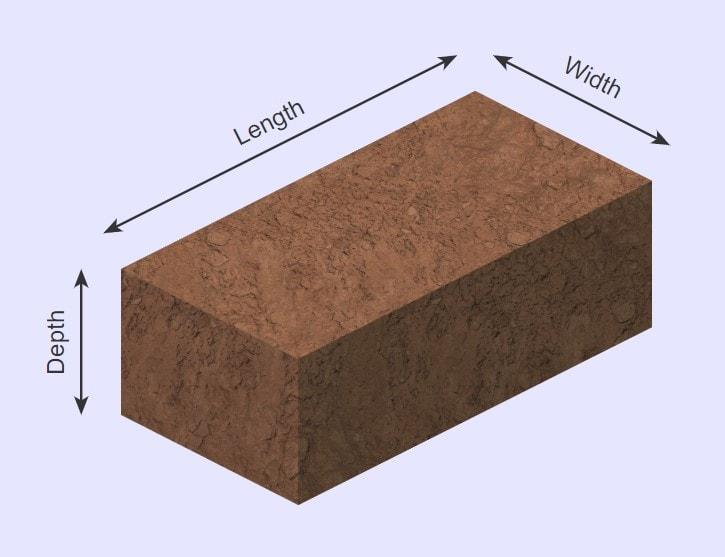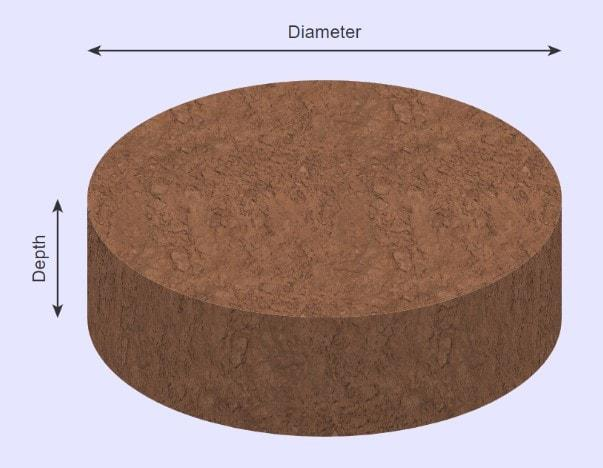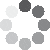Enter parameters Rectangular area
Enter parameters
LENGTH, WIDTH & DEPTH
Length:
ft
• inches (in)
• feet (ft)
• yards (yd)
• centimeters (cm)
• meters (m)
Width:
ft
• inches (in)
• feet (ft)
• yards (yd)
• centimeters (cm)
• meters (m)
Depth:
in
• inches (in)
• feet (ft)
• yards (yd)
• centimeters (cm)
• meters (m)
Price per unit of mass:
t
• pounds (lb)
• US short tons (t)
• imperial-long tons (long t)
• kilograms (kg)
Price per unit of volume:
yd³
• cubic yards (yd³)
• cubic feet (ft³)
• cubic inches (in³)
• cubic centimeters (cm³)
• cubic meters ()Result
Area:
0
ft²
• square feet (ft²)
• square inches (in²)
• square yards (yd²)
• square miles (mi²)
• acres (ac)
• square centimeters (cm²)
• square meters ()
Volume:
0
yd³
• cubic yards (yd³)
• cubic feet (ft³)
• cubic inches (in³)
• cubic centimeters (cm³)
• cubic meters ()
Weight:
0
t
• pounds (lb)
• US short tons (t)
• imperial-long tons (long t)
• kilograms (kg)
Cost:
$Enter parameters Diameter: ft • inches (in) • feet (ft) • yards (yd) • centimeters (cm) • meters (m) Depth: in • inches (in) • feet (ft) • yards (yd) • centimeters (cm) • meters (m) Price per unit of mass: t • pounds (lb) • US short tons (t) • imperial-long tons (long t) • kilograms (kg) Price per unit of volume: yd³ • cubic yards (yd³) • cubic feet (ft³) • cubic inches (in³) • cubic centimeters (cm³) • cubic meters ()Result Area: 0 ft² • square feet (ft²) • square inches (in²) • square yards (yd²) • square miles (mi²) • acres (ac) • square centimeters (cm²) • square meters () Volume: 0 yd³ • cubic yards (yd³) • cubic feet (ft³) • cubic inches (in³) • cubic centimeters (cm³) • cubic meters () Weight: 0 t • pounds (lb) • US short tons (t) • imperial-long tons (long t) • kilograms (kg) Cost:$(5 votes, average: 5.00 out of 5)Loading...
Real-time graphics. Make the calculations and see the changes.
Real-time graphics. Make the calculations and see the changes.Contents:

## How Much Loam Do I Need?

Figuring out how much loam you will need is the first step in preparing for a better lawn or any landscaping project.

Many people find it very difficult to judge by eye exactly how much a pile of soil can cover.

It is also tricky to look at an area of land and to estimate how much soil is required.

The Loam Calculator is a handy tool you can use to calculate the volume of loam required for your project, and if you know the cost per unit of mass, then you know the total cost as well.

Use our Loam Calculator to help you predict how much loam you will need.

This calculator, coupled with a little preparation, can ensure full coverage of your lawn.

## Loam Calculator formula

When you enter your measurements in our calculator, it calculates the volume of loam you will need. Put simply, this is the amount of space the soil will occupy.

### Rectangular AreaThe total volume of a rectangular prism is calculated by:

$$Volume = Length \times Width \times Depth$$

### Circular AreaThe total volume of a circular prism is calculated by:

$$Volume = \pi\ \times \biggl({Diameter \over 2}\biggr)^2 \times Depth$$

Want to know the best part?

If you have the price of soil per unit mass (e.g., cost per pound) or cost per volume (e.g., cost per cubic feet), you can use values as well.

The calculator will then calculate the total price of the soil you will need to purchase using the following formula:

$$Price = Volume \times Price\;per\;unit\;mass$$

or

$$Price = Volume \times Price\;per\;unit\;volume$$

## Worked Example

• Rectangular Area with Price Per Unit Mass

Let’s say I want to fill a rectangular lawn that is 2 ft wide, 3 ft long and 10 inches (0.83 ft) deep.

Let’s also say that the selected loam is sold for $0.1 per pound. The calculator would perform the following calculation: $$Volume = Length \times Width \times Depth = 3 \times 2 \times 0.83 = 5 ft^3$$ I would then enter the price of 0.1$/pound and calculate the cost at $44.50. • Circular Area with Price Per Unit Volume Let’s say I have a circular lawn with a diameter of 12 feet at a depth of 3 feet. Let’s also say that loam costs$0.1 per cubic feet purchased.

The calculator would do the following calculation:

$$Area = \pi\ \times \biggl({Diameter \over 2}\biggr)^2 = \pi\ \times \biggl({12 \over 2}\biggr)^2 = 113.097 ft^2$$

$$Volume = \pi\ \times \biggl({Diameter \over 2}\biggr)^2 \times Depth = 113.097 \times 3 = 339.292 ft^3$$

I would then enter the price per unit volume of 0.1 $/cubic feet and calculate the cost at$33.93.

## What if my measurements aren’t in feet and pounds?

Note that there are multiple units of measure that you can use.

You can enter your measurements of length in inches (in), feet (ft), yards (yd), centimeters (cm) or meters (m). There are also multiple options for mass and volume.

Our calculator does all the conversions for you. Simply select the correct unit in the drop-down areas to convert into a different available unit of measurement.

The calculator will then convert the units using pre-programmed formulae. For example:

$$Volume\;in\;Cubic\;Yards\;(yd^3) = Volume\;in\;Feet\;(ft^3)\;/\;27$$

$$Volume\;in\;Cubic\;Meters\;(m^3) = Volume\;in\;Feet\;(ft^3) × 0.0283$$

It’s that easy!

Embed this calculator on your site!Add live graphics
Copied to clipboard! Preview